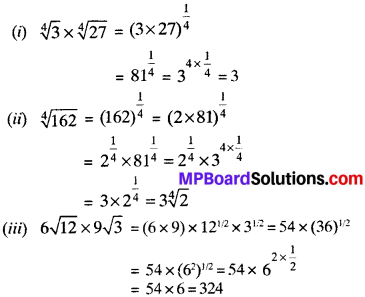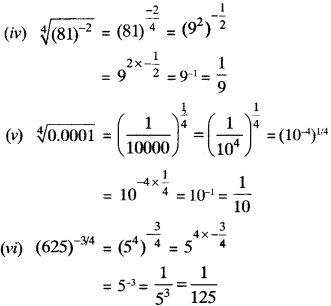## MP Board Class 9th Maths Solutions Chapter 1 Number Systems Ex 1.5

Question 1.
Classify the following numbers as rational or irrational –

1. 2 – √5
2. (3 + $$\sqrt{23}$$ ) – $$\sqrt{23}$$
3. $$\frac { 2\sqrt { 7 } }{ 7\sqrt { 7 } }$$
4. $$\frac{1}{sqrt { 2 }}$$

Solution:

1. 2 – $$\sqrt{5}$$ is an irrational number
2. (3 + $$\sqrt{23}$$ ) – $$\sqrt{23}$$ = 3 is a rational number
3. $$\frac { 2\sqrt { 7 } }{ 7\sqrt { 7 } }$$ = $$\frac{2}{7}$$ is a rational number
4. $$\frac{1}{\sqrt { 2 }}$$ = is an irrational number
5. 2π is an irrational number.Question 2.
Simplify each of the following expressions –

1. (3 + $$\sqrt{3}$$ ) (2 +$$\sqrt{2}$$)
2. (3 + $$\sqrt{3}$$ ) (3 – $$\sqrt{3}$$)
3. ($$\sqrt{5}$$ + $$\sqrt{2}$$)2
4. ($$\sqrt{5}$$ – $$\sqrt{2}$$) ($$\sqrt{5}$$ + $$\sqrt{2}$$)

Solution:
1. (3 + $$\sqrt{3}$$ ) (2 +$$\sqrt{2}$$)
= 6 + 3$$\sqrt{2}$$ + 2$$\sqrt{3}$$ + $$\sqrt{6}$$

2. (3 + $$\sqrt{3}$$) (3 – $$\sqrt{3}$$)
= (3)2 – ($$\sqrt{3}$$ )2 = 9 – 3 = 6

3. ($$\sqrt{5}$$ + $$\sqrt{2}$$ )2
= ($$\sqrt{5}$$)2 + 2$$\sqrt{5}$$ x $$\sqrt{2}$$ + ($$\sqrt{2}$$)2
= 5 + 2$$\sqrt{10}$$ + 2 = 7 + 2$$\sqrt{10}$$

4. ($$\sqrt{5}$$ – $$\sqrt{2}$$) ($$\sqrt{5}$$ + $$\sqrt{2}$$)
= ($$\sqrt{5}$$)2 – ($$\sqrt{2}$$)2 = 5 – 2 = 3

Question 3.
Recall, 7t is defined as the ratio of the circumference (say c) of a circle to its diameter (say d). That is, π = $$\frac{c}{d}$$. This seems to contradiet the fact n is irrational. How will you resolve this contradiction?
Solution:
No contradiction will be there. Whenever we measure any length with any device, we only get an approx, rational value and so cannot realise that either c or d is irrational.Question 4.
Represent $$\sqrt{9.3}$$ on the number line.
Solution:
Steps:

1. Draw a line segment AC = 9.3 cm and extend it to B such that CB – 1 cm.
2. Draw the perpendicular bisector of AS and mark the mid point O of AB.
3. With O as centre and OB as radius, draw a semicircle.
4. At point C, draw perpendicular CD which intersect the semicircle at D such that CD = $$\sqrt{9.3}$$.
5. With C as centre and CD as radius, draw an arc which intersect AB produced at E.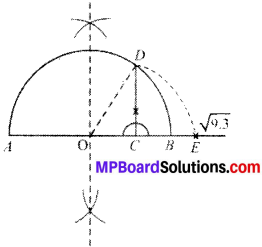Proof:
OA = OB = $$\frac{10.3}{2}$$ = 5.15cm
OD = 5.15 cm
OC = OB – BC = 5.15 – 1 = 4.15 cm
CD = $$\sqrt { OD^{ 2 }-OC^{ 2 } }$$
= $$\sqrt{9.3}$$ cm

Question 5.
Rationalise the denominators of the following:

1. $$\frac{1}{\sqrt { 7 }}$$
2. $$\frac{1}{\sqrt{7}-\sqrt{6}}$$
3. $$\frac{1}{\sqrt{5}+\sqrt{2}}$$
4. $$\frac{1}{\sqrt{7}-2}$$

Solution:
1. $$\frac{1}{\sqrt { 7 }}$$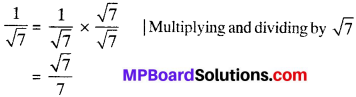2. $$\frac{1}{\sqrt{7}-\sqrt{6}}$$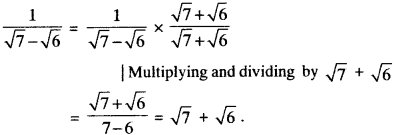3. $$\frac{1}{\sqrt{5}+\sqrt{2}}$$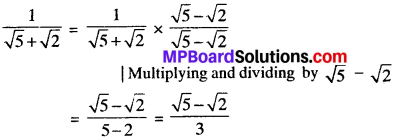4. $$\frac{1}{\sqrt{7}-2}$$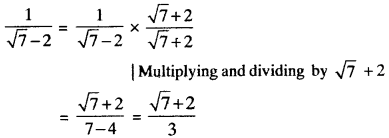Laws of Exponents for real numbers:
If a, n and m are natural numbers, then

1. am x an = am+n
2. (am)n = amn
3. $$\frac { a^{ m } }{ b^{ n } }$$ where m > n
4. am x bm – (ab)m

Example 1.

1. 23 x 24 = 23+4 = 27
2. (32)3 = 32×3 = 36
3. $$\frac { 5^{ 5 } }{ 5^{ 2 } }$$ = 55-2 = 53
4. 23 x 33 = (2 x 3)3 = 63Example 2.
1. 6$$\frac{2}{5}$$ x 6$$\frac{3}{5}$$
Solution:
6$$\frac{2}{5}$$ x 6$$\frac{3}{5}$$ = 6$$\frac{5}{5}$$ = 6

2. 6$$\frac{1}{2}$$ x 7$$\frac{1}{2}$$
Solution:
6$$\frac{1}{2}$$ x 7$$\frac{1}{2}$$ = (6 x 7)$$\frac{1}{2}$$ = 42$$\frac{1}{2}$$

3. $$\frac{5^{6 / 7}}{5^{2 / 3}}$$
Solution: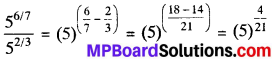Example 3.
Simplify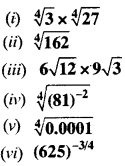Solution: